### Focus-directrix definition of the parabola

The Greeks defined the parabola using the notion of a locus. A locus is a set of points satisfying a given condition. These points will generally lie on some curve. For example, the circle with centre $$O$$ and radius $$r$$ is the locus of a point $$P$$ moving so that its distance from the point $$O$$ is always equal to $$r$$.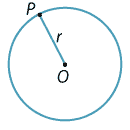Detailed description of diagram

The locus definition of the parabola is only slightly more complicated. It is very important and gives us a new way of viewing the parabola. One of the many applications of this is the refection principle, which we will look at in a later section.

We fix a point in the plane, which we will call the focus, and we fix a line (not through the focus), which we will call the directrix. It is easiest to take the directrix parallel to the $$x$$-axis and choose the origin so that it is equidistant from the focus and directrix. Thus, we will take the focus at $$S(0,a)$$ and the directrix with equation $$y=-a$$, where $$a > 0$$. This is as shown in the following diagram.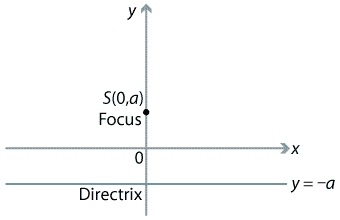Detailed description of diagram

We now look at the locus of a point moving so that its distance from the focus is equal to its perpendicular distance to the directrix. Let $$P(x,y)$$ be a point on the locus. Our aim is to find the equation governing the coordinates $$x$$ and $$y$$ of $$P$$.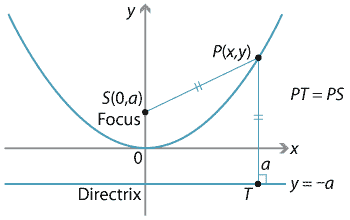Detailed description of diagram

The distance $$PS$$ is equal to $$\sqrt{x^2 + (y-a)^2}$$, and we can see from the diagram that the perpendicular distance $$PT$$ of $$P$$ to the directrix is simply $$y+a$$.

Since $$PS=PT$$, we can square each length and equate, giving

$x^2+(y-a)^2 = (y+a)^2 \ \implies \ x^2=4ay.$

The last equation may also be written as

$y= \dfrac{x^2}{4a}$

and so we see that it is the equation of a parabola with vertex at the origin.

The positive number $$a$$ is called the focal length of the parabola.

Any parabola of the form $$y=Ax^2+Bx+C$$ can be put into the standard form

$(x-p)^2 = \pm 4a(y-q),$

with $$a > 0$$, where $$(p,q)$$ is the vertex and $$a$$ is the focal length. When a parabola is in this standard form, we can easily read off its vertex, focus and directrix.

#### Example

Find the vertex, focal length, focus and directrix for the parabola $$y=x^2-4x+3$$.

#### Solution

We complete the square and rearrange as follows:

\begin{align*} y = x^2-4x+3 \ &\implies \ y=(x-2)^2-1 \\ \ &\implies \ (x-2)^2= 4\times \dfrac{1}{4}(y+1). \end{align*}

Hence the vertex is $$(2,-1)$$ and the focal length is $$\dfrac{1}{4}$$.

The focus is then $$(2, -\dfrac{3}{4})$$ and the equation of the directrix is $$y=-\dfrac{5}{4}$$.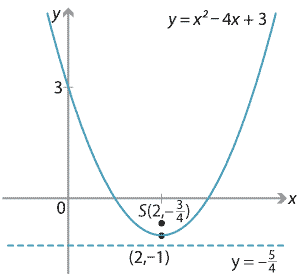Detailed description of diagram

A parabola may also have its directrix parallel to the $$y$$-axis and then the standard form is $$(y-p)^2 = \pm 4a(x-q)$$, with $$a > 0$$.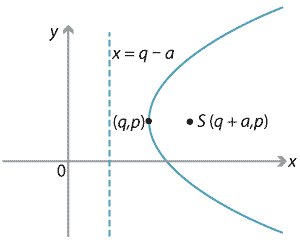Detailed description of diagram

##### Exercise 13

Put the parabola $$y^2-2y= 4x-5$$ into the above standard form (for a directrix parallel to the $$y$$-axis) and thus find its vertex, focus and directrix, and sketch its graph.

Next page - Content - Parametric equations of a parabola